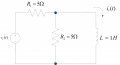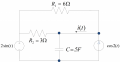# Circuit analysis with complex exponential time functions. Help plz!!

#### flusteredStudent

Joined Sep 8, 2014
2The circuit above has a single input (complex exponential time func. that is not switched with no initial conditions.)
Vs(t) = 10*e^(j 3t)
Calculate the current through the inductor i l(t).

Also...

Calculate current for i(t) (below).Step by step explanation would be amazing and very very helpful/needed

#### MrAl

Joined Jun 17, 2014
7,145
Hi,

To start, did you try using Euler's Formula for the complex exponential yet?

#### flusteredStudent

Joined Sep 8, 2014
2
Not yet, would a s-domain transform be an ideal strategy?

#### MrAl

Joined Jun 17, 2014
7,145
Hi,

Well for the first circuit there, if the complex exponential was converted then yes it seems that you could work in the s domain and use superposition. Try that